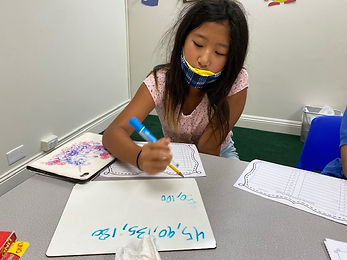## Ms. Dawn

### Target 1​

###### Lesson Type:

Continuation

Number Operation

:

Fractional Numbers

Convert between fractions, decimals, and percentages.

###### 1:

Describe the relationship between fractions, decimals, and percentages.

###### 2:

Convert fractions to decimals and decimals to fractions.

4th

###### Vocabulary:

Fraction, Decimal, Percentage, Convert, Denominator, Numerator

Activities:

1. Students verbally described how fractions, decimals and percents are related.

2. Students were given a set of animals and needed to write the fraction it represented. Then, convert it to decimals and percentages.

3. Students used dominoes to create a fraction and then converted it to decimals and percentages using their strategies they learned.### Home Exploration

###### Guiding Questions:## Absent Students:

### Target 2

:

###### 1:

Define Least Common Multiple (LCM).

###### 2:

List the LCM for two whole numbers.

###### 3:

Understand that different numbers can share factors.

4th

###### Vocabulary:

Least Common Multiple, Multiple, Venn Diagram

Activities:

1. Discussed the definition of Least Common Multiple. We used a Venn Diagram as a practice example to compare two numbers and found the Least Common Multiple.

2. Students were given task cards with two different numbers and they needed to find the Least Common Multiple. They used their whiteboards to list the multiples of each number to help them find the Least Common Multiple.### Home Exploration

###### Guiding Questions:### Target 3

:

###### Vocabulary:

Activities:### Home Exploration